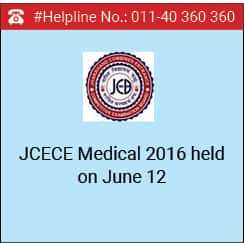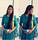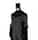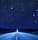Following the implementation of the NEET ordinance allowing states to conduct their own medical entrance exam for the current academic session, the Jharkhand Combined Entrance Competitive Examination Board (JCECEB) has conducted JCECE Medical 2016 on June 12, 2016 in the pen and paper based mode. The entrance exam was conducted for admissions to MBBS and BDS programmes in the State.

The question paper consisted of 150 multiple choice questions from Physics, Chemistry and Biology. Each question consisted of four options, out of which, candidates had to choose the most appropriate answer.

The result of JCECE Medical 2016 will be declared in the month of June, 2016. According to the officials, candidates have to score a minimum of 50% marks in JCECE Medical 2016 irrespective of their caste categories in order to qualify the entrance exam.

The counselling of JCECE Medical 2016 is scheduled to be conducted, tentatively, in the month of July. Allotment of MBBS and BDS seats will be done strictly on the basis of the candidate’s rank in state merit list.

Admissions to 85% state quota seats of MBBS and BDS programmes in the government medical and dental colleges of Jharkhand will be given on the basis of JCECE Medical 2016. However, admissions to 15% All India quota seats will be given on the basis of NEET 2016 result, scheduled to be declared on August 17, 2016.

Stay tuned to www.medicine.careers360.com for more news and information on JCECE Medical

### Applications Open NowUPES - School of Health Sciences
View All Application Forms

## Questions related to NATA

Showing 3319 out of 3319 Questions
7 Views

## i have a experince 4 years in interior designer and i have a degree in interior designer from punjab technical university jalandhar can i do nata exam is eligibale and what is the processSharon Rose Student Expert 9th Nov, 2019Hello Aman,

The eligibility criteria for NATA is given below

• A candidate must have passed 10+2 with atleast 50% aggregate and must have studied Mathematics, Physics and Chemistry as compulsory subjects.
• There is no age limit.
• Diploma holders are not eligible.

So, if you want to pursur B.Arch course then the admission is based on 10+2 and merit in NATA.So your graduation in interior design and experience is not considered while seeking admission in B.arch through NATA.

And when the notification is released for NATA 2020 you can apply for it by filling the application form and exam will be conducted, you need to qualify in that exam and the admission is based on merit in NATA exam.
You may see the detailed eligibility criteria by using the link below

I hope this is helpful to you.
1 View

## how is the nata question paper based on

Ronak Gala Student Expert 5th Nov, 2019Nata exam is based upon different subjects.

I'll guide you with the subjects and to know their syllabus in detail, I'll provide you with the link at the end, so that you can get the complete syllabus ofntheese given subjects.

So the first subject is Mathematics.

The second one is General Aptitude.

The last one is the Drawing Test.

• There will be 2 parts in the exam- part A of 60 minutes duration while Part B of 120 minutes duration

• Part A will include choice questions (MCQs) and will be conducted online. Meanwhile, Part B will be Drawing test to be answered on A4 size papers

• Part A comprises Mathematics (20 Questions) and General Aptitude (40 Questions). Each question will be of 2 marks.

• Part B will be offline based and include 2 questions each carrying 40 marks

• There is no negative marking

Best wishes. Thank you.

5 Views

## when starting exam of NATA in our countryNilutpol Student Expert 4th Nov, 2019Hello,
National Aptitude Test in Architecture (NATA) is a national level undergraduate exam which is conducted by Council of Architecture (COA) for admission to 5-year B.Arch programme.
This year NATA exam will be conducted twice a year. Once in April and then in July.
The application form for April session will be out in the month of January. So just keep an eye on the official website.

https://engineering.careers360.com/exams/nata
Hope it helps
16 Views

## I want to join architecture college .. I need help in NATA exam can any one help meAditi Amrendra Student Expert 31st Oct, 2019Hello,

The most important thing is syllabus so first go through it.
NATA Syllabus 2019 for Mathematics
Definitions of A. P. and G.P.; General term; Summation of first n-terms of series n, n2,n 3 ;
Arithmetic/Geometric series, A.M., G.M. and their relation; Infinite G.P. series and its sum.
Logarithms
Definition; General properties; Change of base.
Matrices
Concepts of m x n (m 3, n 3) real matrices, operations of addition, scalar multiplication and
multiplication of matrices. Transpose of a matrix. Determinant of a square matrix. Properties of determinants
(statement only). Minor, cofactor and adjoint of a matrix. Nonsingular matrix. Inverse of a matrix. Finding area
of a triangle. Solutions of system of linear equations. (Not more than 3 variables).
Trigonometry
Trigonometric functions, addition and subtraction formulae, formulae involving multiple and
submultiple angles, general solution of trigonometric equations. Properties of triangles, inverse trigonometric
functions and their properties.
Coordinate geometry
Distance formula, section formula, area of a triangle, condition of collinearity of three
points in a plane. Polar coordinates, transformation from Cartesian to polar coordinates and vice versa. Parallel
transformation of axes, concept of locus, elementary locus problems. Slope of a line. Equation of lines in
different forms, angle between two lines. Condition of perpendicularity and parallelism of two lines. Distance
of a point from a line. Distance between two parallel lines. Lines through the point of intersection of two lines.
Equation of a circle with a given center and radius. Condition that a general equation of second degree in x, y
may represent a circle. Equation of a circle in terms of endpoints of a diameter . Equation of tangent, normal
and chord. Parametric equation of a circle. Intersection of a line with a circle. Equation of common chord of
two intersecting circles.
3-Dimensional Co-ordinate geometry
Direction cosines and direction ratios, distance between two points
and section formula, equation of a straight line, equation of a plane, distance of a point from a plane.
Theory of Calculus
Functions, composition of two functions and inverse of a function, limit, continuity,
derivative, chain rule, derivative of implicit functions and functions defined parametrically. Integration as a
reverse process of differentiation, indefinite integral of standard functions. Integration by parts. Integration
by substitution and partial fraction. Definite integral as a limit of a sum with equal subdivisions. Fundamental
theorem of integral calculus and its applications. Properties of definite integrals. Formation of ordinary
differential equations, solution of homogeneous differential equations, separation of variables method, linear
first order differential equations.
Application of Calculus
Tangents and normals, conditions of tangency. Determination of monotonicity,
maxima and minima. Differential coefficient as a measure of rate. Motion in a straight line with constant
acceleration. Geometric interpretation of definite integral as area, calculation of area bounded by elementary
curves and Straight lines. Area of the region included between two elementary curves.
Permutation and combination
Permutation of n different things taken r at a time (r n). Permutation of n
things not all different. Permutation with repetitions (circular permutation excluded). Combinations of n
different things taken r at a time (r n). Combination of n things not all different. Basic properties. Problems
involving both permutations and combinations.
Statistics and Probability
Measure of dispersion, mean, variance and standard deviation, frequency
distribution. Addition and multiplication rules of probability, conditional probability and Bayes Theorem,
independence of events, repeated independent trails and Binomial distribution.

NATA 2019 Syllabus for General Aptitude

General
Objects, texture related to architecture and built environment. Interpretation of pictorial compositions,
Visualizing three-dimensional objects from two-dimensional drawing. Visualizing different sides of 3D objects.
Analytical reasoning, mental ability (visual, numerical and verbal), General awareness of national/
international architects and famous architectural creations.
Mathematical reasoning
Statements, logical operations like and, or, if and only if, implies, implied by.
Understanding of tautology, converse, contradiction and contrapositive.
Sets and Relations
Idea of sets, subsets, power set, complement, union, intersection and difference of sets, Venn diagram, De Morgan's Laws, Relation and its properties. Equivalence relation definition and elementary examples.

NATA Syllabus 2019 for Drawing Test

Drawing Test
Syllabus
Understanding of scale and proportion of objects, geometric composition, shape, building forms and elements, aesthetics, colour texture, harmony and contrast. Conceptualization and Visualization through structuring objects in memory. Drawing of patterns - both geometrical and abstract. Form transformations in 2D and 3D like union, subtraction, rotation, surfaces and volumes. Generating plan, elevation and 3D views of objects. Creating 2D and 3D compositions using given shape and forms. Perspective drawing, Sketching of urbanscape and landscape, Common day-to-day life objects like furniture, equipment etc., from memory.

You cant really prepare for NATA.This exam is designed in such a way that it tests a lot of thing of a candidate.It requires a basic sense of aptitude knowledge. The drawing questions tests your creative imagination along with your sense of perspective.

Past year papers can help you a lot.Just go through then and observe types of questions that are being asked and prepare accordingly.

Watch online videos if you are not enrolled in amy coaching.

In order to perform well in this exam you will have to dive in it completely.

I hope this helps.
14 Views

## can i use my marks from NATA 2019 July to get admission in Manipal university in 2020. As according to my knowledge NATA result is valid for 1 year and the admission process of Manipal University takes place at the month of May and June so will i be able yo use that makrs. please help...Nilutpol Student Expert 31st Oct, 2019Hello,
NATA Score is valid for a period of 24 months (2 years) from the date a candidate appears for NATA exam. If 24 months period is over, aspirants have to register for next nATA examination and will have to secure a valid NATA Score/ Rank.
Since you have appeared for NATA in the year 2019, so you can appear for admission inthe academic year 2020 for various institution for B Arch and B.plan.
Hope it helps
Top
The question have been saved in answer later, you can access it from your profile anytime. Access now